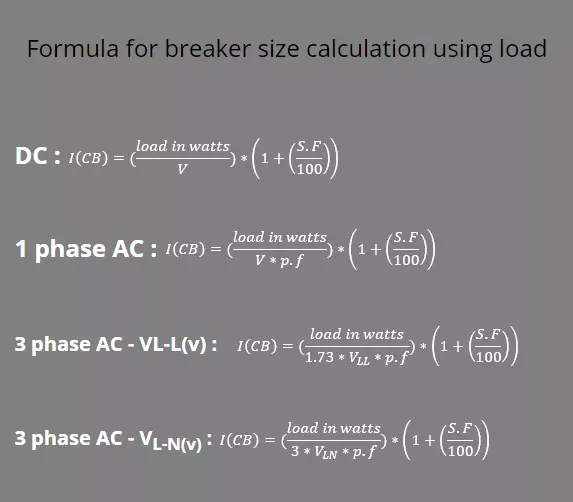(206) 687-4009 | [email protected]

# Breaker Size Calculator

As equipment are designed to work at an allowed current. If equipment current exceeds the allowed current for a long duration it may damage equipment insulation and may also catch fire. In order to protect equipment from overload and short circuit, breakers are used, which disconnect the equipment after a certain delay if any fault or overloading condition occurs.

The desired breaker for power system equipment are selected by calculation commonly known as breaker sizing.

Choose the method
Phase Type
S.F
%
Power
Voltage
VoltsChoose the method

S.F
%
Current
amps## Why accurate breaker sizing is required?

For better reliability purpose it is important to properly calculate breaker size.

• Overly size breaker will not be tripping under low fault or high overloading condition, effecting dependability of system
• Under size breaker will issue trip under normal condition, effecting security of system

## What is safety factor (S.F)?

Some equipment allows to work for a certain overload condition for certain time. Breaker sizing are done in consideration of overload condition for the better security purpose. For example: a motor has a rated current of 100 A but can work at 125 A for one hour, this 25% increase in current as compared to rated current is known as safety factor.

Common loads and their safety factor:

Air conditioner and heat pump 75%
Welders 100%
Motors 25%

Breaker sizing calculator parameter:

• Choose the method: provide load (in kilowatts or watts) and current (in amps)
• If current selected: rated current of equipment and required safety factor (S.F) to be entered

For option: For DC, 1∅ AC and 3∅ AC.

For DC circuits: voltage (in volts), power (in watts or kilowatts) and safety factor (S.F) (in percentage) are required

For AC circuits: voltage (in volts), power (in watts or kilowatts), power factor (P.F) (in units or percentage) and safety factor (S.F) are required.

### Steps for breaker sizing calculator:

When current is given:

The formula for breaker current I (CB) when equipment rated current I (A) is provided:

I(C.B) = I(A) * (1 +
S.F / 100
)

For DC circuit:

The formula for DC circuits is given below.

I(C.B) =
power in watts / V
* (1 +
S.F / 100
)

For 1 phase AC circuit:

The formula for single phase AC circuit is same as DC with an addition of power factor (p.f) which is given as:

I(C.B) =
power in watts / V * p.f
* (1 +
S.F / 100
)

For 3 phase AC circuits:

The formula for three phase AC circuit is same as two phase AC circuit but instead of 2 we use square root 3 (~1.73) when voltage is expressed in terms of line to line (Vll) which is given as:

I(C.B) =
power in watts / 1.73 * vLL * p.f
* (1 +
S.F / 100
)

When voltage is expressed in terms of line to neutral then we use 3 instead of 1.73.

I(C.B) =
power in watts / 3 * vLN * p.f
* (1 +
S.F / 100
)

## Note

In the above formulas:

• Power factor (p.f) is given in form of unit, ranging from 0 to 1 (for example: 0.8, 0.9). If p.f is expressed in terms of percentage then it is first converted into units by dividing percentage power factor by 100 and then its value is given in the formula.
• Power here in this formula is expressed in watts, if user define it in form of kilowatts then first it is converted into watts by dividing Kilowatts with 1000 and then its value is given in the formula.
• Breaker comes in some standard sizes. Sometimes the calculated breaker size is not available in market. So you can use the nearby rating ampere breaker.For example: breaker ampere from calculation is 45 amps and in market a 50 amps breaker is available. So we can use 50 amps breaker

## Solved Example:

### When current is given:

Consider a system whose rated current is provided

Given:

Rated current=I(A) = 20 Amps

Safety factor (S.F) = 25%

Required:

Breaker current=I(CB) =? (Amps)

Solution:

I(C.B) = 20 * (1 +
25 / 100
) 25 Amps

### When load (watts) is given:

For single phase system:

Consider a single phase AC system that has the following data:

Given:

Voltage =230 V

Power= 1.5 KW or 1500 W

Safety factor (S.F) =25%

Required:

Breaker current=I(CB) =? (Amps)

Solution:

I(C.B) =
1500 / 230 * 0.9
* (1 +
25 / 100
) 9.05 Amps~10 Amps

For 3 phase system:

Consider a three phase system that has the following data:

Given:

Voltage (line to line) =480 V

Power = 20 KW or 20,000 W

Power factor (p.f) =0.9

Safety factor (S.F) =20%

Required:

Breaker current=I(CB) =? (Amps)

Solution:

From three phase AC circuit formula:

I =
20,000 / 1.73 * 480 * 0.9
*(1 +
20 / 100
) 32.07 Amps~33 Amps

If we change voltage from line to line to line to neutral, for example: V (line to neutral) =277.13 V

Then we will be calculating it using formula of 3 phase AC circuit when voltage is given as line to neutral that is:

I =
20,000 / 3 * 277.13 * 0.9
* (1 +
20 / 100
) 32.07 Amps~33 Amps